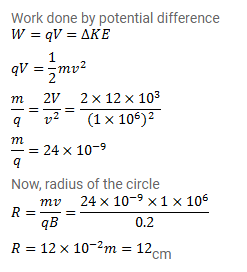# A charged particle is accelerated through a potential difference

Question:

A charged particle is accelerated through a potential difference of $12 \mathrm{kV}$ and acquires a speed of $1.0 \times 10^{6} \mathrm{~m} / \mathrm{s}$. It is then injected perpendicularly into a magnetic field of strength $0.2 \mathrm{~T}$. Find the radius of the circle described by it.

Solution: